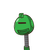# mx +5y -11 = 0 and 14x + 10y – 22=0 if these simulatianeous equations are parallel to each other then find value of m​

mx +5y -11 = 0 and 14x + 10y – 22=0 if these simulatianeous equations are parallel to each other then find value of m​

### 1 thought on “mx +5y -11 = 0 and 14x + 10y – 22=0 if these simulatianeous equations are parallel to each other then find value of m​”

1.m = 7/2

Step-by-step explanation:

equation 1 , mx + 5y = 11

5y = -mx +11

y = (-m/5) + 11

now comparing it with y = (slope)x + c

so , slope of line 1 = -m /5

similarly slope of 2 line

y = (-14/10)x +22

slope = -14/20

now if both lines are parallel then there slopes are equal so,

-m/5 = -14/20

m = 14/4

m = 7/2